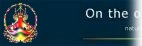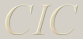Table of contents An important message What CIC can do An example using the comparison tables Bug report   An example using the comparison tables   A basic loan First click 'Loan repayment - find payment amount'. Let's say the original loan is \$300,000, it is designed to be active for 30 years, the interest rate per year is 10.25%, the interest is calculated monthly (that is, 12 times a year), and the repayments take place monthly (12 times a year). After entering these values click Calculate and you get - Repayment needed (for every month): \$2,686.74 Total interest paid: \$667,224.64 Total cost of the loan: \$967,224.64 At that point the Year-by-year table shows the balance of the loan and the interest paid at each year during the life of the loan (based on the entire loan arrangement). Notice that only after the 20th year you start decreasing the principal. Click Create table to view a summary of the year-by-year total costs incurred if you were to roll over the original loan into a new one after any one of those years, as well as the combined costs of both.   Roll-over loan Let's assume you consider rolling the loan over into another one after 5 years, in other words, after 60 repayments have been made. Enter '60' next to 'How many repayments have been made', click Calculate and you get \$161,204.11 as the cost of the loan so far and \$290,315.77 as the remaining balance of the original loan, which forms the starting point of that new loan. Open another window by clicking Go! next to "Loan repayment - find payment amount" again and paste the amount of \$290,315.77 into the field next to 'Initial loan' in the new window. Assuming the other figures are the same enter those figures into the other fields (ie, '30', '10.25', '12', '12'). You'll get the following results - Repayment needed (for every month): \$2,600.01 Total interest paid: \$645,686.12 Total cost of the loan: \$936,001.89 The Year-by-year table shows the changing balance and interest paid for each year of the loan.   Using memory store If at that point you want to know the combined costs of the first loan after those 5 years plus what the costs are of the new loan, use the memory store to add the total cost of this loan (\$936,001.89) to the total cost of the original loan after 5 years as shown in the first window (\$161,204.11), and you get \$1,097,206.00. Notice you get the same answer by looking up the first comparison table and checking for the total cost of both loans at the 5-year line, which is \$1,097,205.99 (the minimal difference between the results in the memory store and the comparison table is due to the rounding employed in performing the calculations). If you only want a summary that's all you have to do, otherwise use the second window to get more details, as described above. If you want to work out the results with a different loan period, or a different interest rate, enter the respective figure into its field in the second window and click Calculate again.   Roll-over continued To work through a similar scenario once again enter the number of repayments made on that window and continue. Let's say you consider rolling over that second loan after 8 years (clicking on Create table will show you the summary this time), then there will have been 96 repayments, and therefore the balance of the loan after 8 years will be \$272,544.46. Continue in this fashion by opening another window, and so on. Use tags to differentiate between the various results to help keeping them apart in the memory store. As you walk through the roll-overs, notice that the total cost of the original \$300,000 loan will always be smaller than the total costs of the loans derived from the roll-overs. Although the individual repayments will get smaller as you go along, not only will the time spent in contractual loan arrangements have become longer, the combined costs will have increased substantially. Use more windows if you want to get an idea of what the interest and costs will be like if the interest rate should go up or down in the future.   Pay more than needed CIC can tell you how larger repayments affect the loan. Using the first \$300,000.00 loan example, let's assume you decide to pay \$300.00 on top of the \$2,686.74 needed. Enter '300' next to 'Amount added to repayment needed', click Calculate in the 'Initial loan' section and the total interest paid is now \$385,339.68 and the total cost has been reduced to \$685,339.68. Click Create table to view the comparison table under the new repayments. Notice it stops at year 19 because after that there is no loan derived from the remaining balance left (assuming the same interest rate, the larger repayments have a greater effect on the smaller loan based on the remaining balance). If you now check the cost of the loan after 5 years (60 repayments), click Calculate in the 'Remaining balance...' section and you get \$179,204.11 as the cost of the loan so far, and \$266,930.51 as the balance of the loan. Enter the balance into a new window to see the details and, assuming interest rate etc remained the same, you get \$2,390.57 for the repayment needed, \$593,675.38 for the total interest paid, and \$860,605.89 for the total cost of the loan. Note that these figures are based on the required repayments needed, any additional payments used in the previous scenario are no longer used. Suppose you want to add \$200.00 to the repayment amount. Enter '200' next to 'Amount added to repayment needed', click Calculate in the 'Initial loan' section and the total interest paid will now be \$379,183.53 and the total cost of the loan has become \$646,114.04. Click Create table to view the comparison table. Notice it stops at year 20 because there is no remaining balance left in a roll-over. To confirm this, enter first '240' (ie, 20 years) in the 'How many repayments have been made' field and the balance of the loan will be \$23,324.87. Now change the number of repayments made to '252' (21 years) and you'll get an error message because you have exceeded the life of the loan.   Saving the results Click Copy to clipboard at any time to paste the result into some document or spreadsheet (you may have to increase the page width to view the columns correctly).